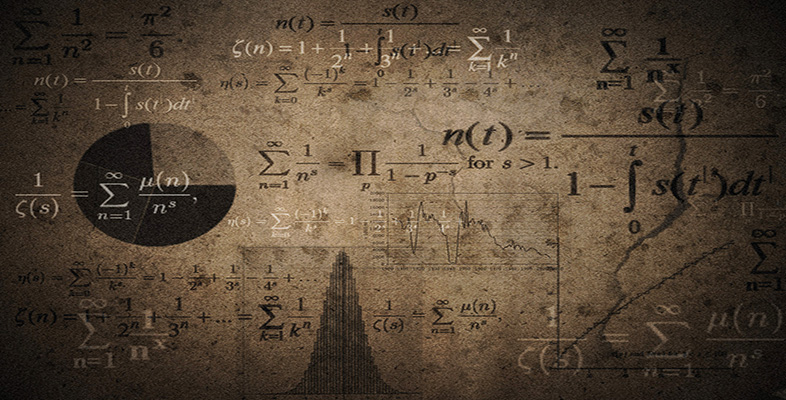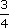Language, notation and formulas

This free course is available to start right now. Review the full course description and key learning outcomes and create an account and enrol if you want a free statement of participation.

Free course

# 2.5.1 Try some yourself

## Activity 5

How would you add the following words to the list:

decimal, fraction, positive, negative.

For each one, give the mathematical meaning and an example of its use.

There are many different correct answers to this question. However, make sure that the meanings you have given are equivalent to the ones below.

Word Mathematical meaning Example of use
Decimal A number expressed in terms of tenths, hundredths, etc. A quarter expressed as a decimal is 0.25.
Fraction One whole number over another. 0.75 expressed as a fraction is.
Positive Greater than zero. 2 is a positive number.
Negative Less than zero. 2 is a negative number.
MU120_4M6